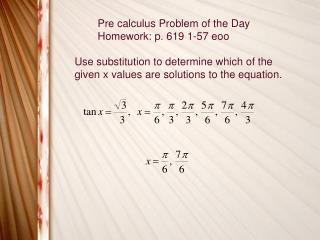# Pre calculus Problem of the Day Homework: p. 619 1-57 eoo - PowerPoint PPT PresentationDownload PresentationPre calculus Problem of the Day Homework: p. 619 1-57 eoo

Pre calculus Problem of the Day Homework: p. 619 1-57 eooDownload Presentation## Pre calculus Problem of the Day Homework: p. 619 1-57 eoo

- - - - - - - - - - - - - - - - - - - - - - - - - - - E N D - - - - - - - - - - - - - - - - - - - - - - - - - - -
##### Presentation Transcript

1. Pre calculus Problem of the Day Homework: p. 619 1-57 eoo Use substitution to determine which of the given x values are solutions to the equation.

2. Solving Trigonometric Equations, Finding all Solutions Where n is any integer. An equivalent answer would be

3. Isolate a function to solve a trigonometric equation.

4. Solve a trigonometric equation by factoring.

5. Solving a trigonmetric equation over an interval. Find all solutions on the interval [ 0, 2¶ ).

6. Pre calculus Problem of the Day Homework: p. 619 63 - 83 odds, 85-109 eoo Solve the following over the interval [ 0, 2¶).